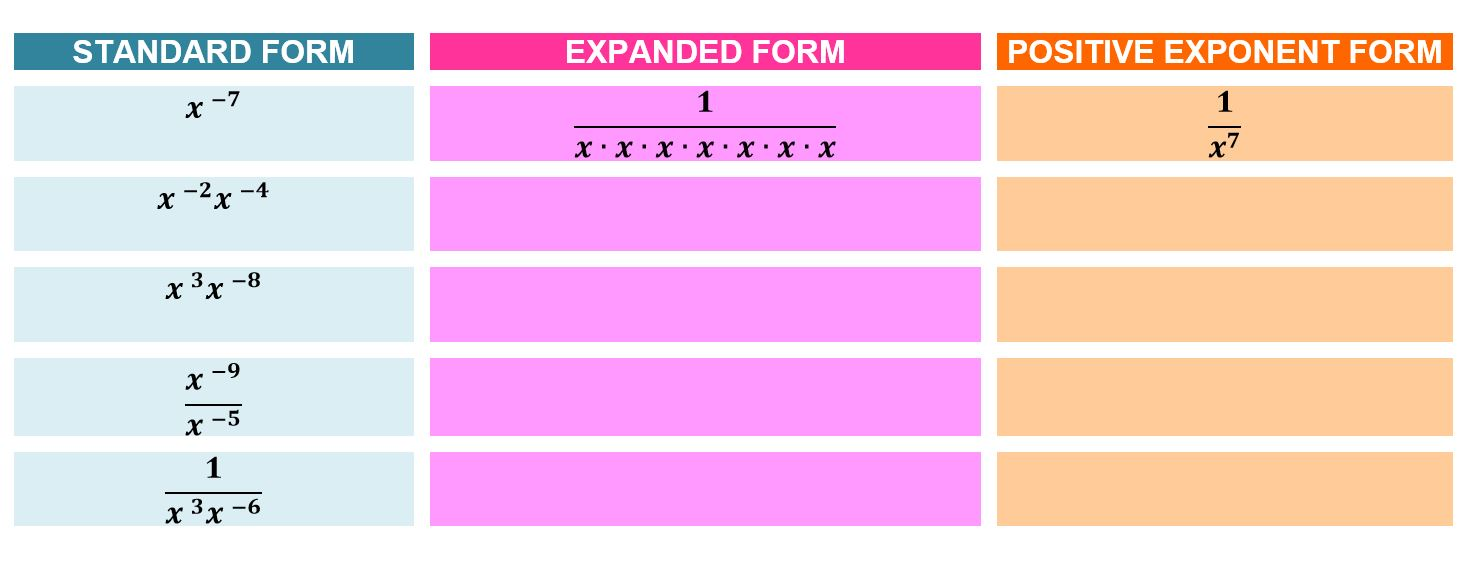# Know and Apply the Properties of Integer Exponents

 Would You Rather Listen to the Lesson?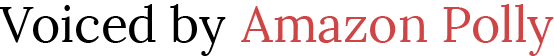In pre-algebra, it’s important for students to know and apply the properties of integer exponents. But before they’re able to do that, a sound understanding of definitions is important. Exponents are those small and mighty numbers that are more powerful than you’d expect.  I always make sure that I start by showing the expanded form of an exponential expression.  This not only reviews what an exponent is and does, but it helps students see patterns and start to learn the laws of exponents.

It’s important to share the different rules and laws of exponents but be careful not to do it too soon. The students can figure the rules out during this lesson and when they do, it has an exponential effect!  If they are just told to memorize some rule, they tend to forget it and not really understand the difference of powers.

To help students envision the meaning of these expressions, I have students model multiplication and division of exponents using small items like the ones listed below:

• algebra tiles
• coins
• donuts
• cheerios
• poker chips
• post-its

Or simply anything I have on hand, just as long as I have plenty of them, because they will pile up quickly. We are dealing with exponential expressions remember? Then I introduce the power of zero and negative exponents.

Once they have a foundation, we play the Integer Exponent Card Game.  It’s a bit like war, but the students each throw 2 cards at a time.  In order to really practice negative exponents, the goal is to throw the smallest product or quotient.  And there’s a catch, if they throw two cards that give them an exponent of zero, they win the round!

## Set up for the Lesson: Know and Apply the Properties of Integer Exponents

1. Have small cups of cheerios or coins, algebra tiles or tokens for each student.  It’s best if they are the same color, because students will be modeling exponents with the same base.
2. Have a deck of cards for each pair of students
3. A Integer Exponent Card Game: Round 1 Product Template and Round 2 Quotient template (one copy per student)
4. Provide each student with the Integer Exponent Card Game Worksheet: this has a game sheet for them to record expressions and their score as well as reflection questions and practice

## Launch the Lesson: Know and Apply the Properties of Integer Exponents

Students should already be familiar with positive exponents, but have them model a positive exponential product and quotient to help introduce the concept of negative exponents.  You can do this with the cheerios, tokens, donuts, or other manipulative.

Use the guide below in launching the lesson:

## Product of Exponents

• Emphasize that one donut stands for x
• Tell them that when the donuts are next to each other, it means to multiply.
• So, for example:doesn’t mean 4x, it means or x ∙ x ∙ x ∙ x or x⁴
• This is a great time to make sure your students understand what exponent means.
• Then ask them to model x⁴x³.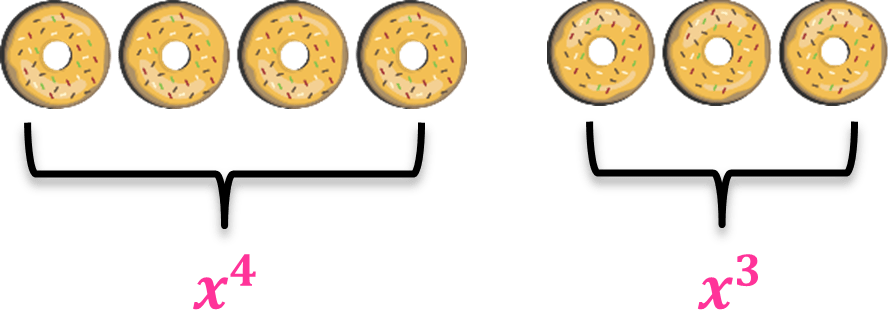• Go around and watch how they model it. They should understand that x⁴ is x written four times, and so is x written three times.
• So x⁴x³ is the same as (x ∙ x ∙ x ∙ x) (x ∙ x ∙ x) or  x ∙ x ∙ x ∙ x ∙ x ∙ x ∙ x, where x is written seven times.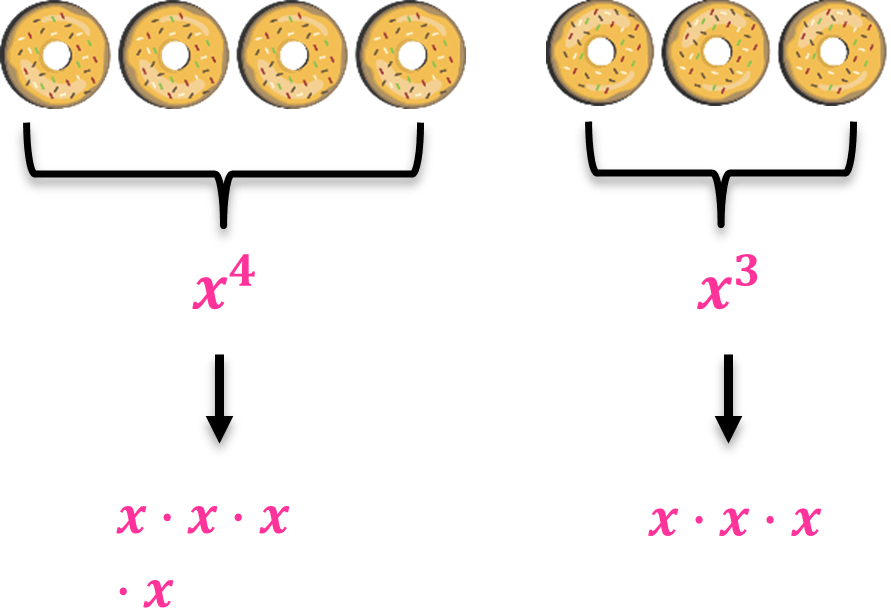• They might have 7 cheerios, tokens, donuts, or any manipulative in front of them.
• So ask “How could we write this product?” They should recognize that x is written 7 times, so the product would be x
• They may arrange the manipulative in a row, same as the image above.
• Have the partners talk about what they did to get 7. They should come up with things like “we added them together” or “we combined them.”
• So, even though they were multiplying the expressions, they see that they added the exponents.

## Quotient of Exponents

Now, let’s move on to division of exponents. Note to the designer: Place quotients as fractions with a top and a bottom, like this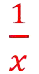(not a backslash)

• Emphasize that one donut stands for x
• Let themdivide by. They can even put it on a division/fraction template. Refer to the image below.• Ask: “What is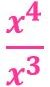?
• Show how the donuts are cancelled out and how we are left with only one donut on top.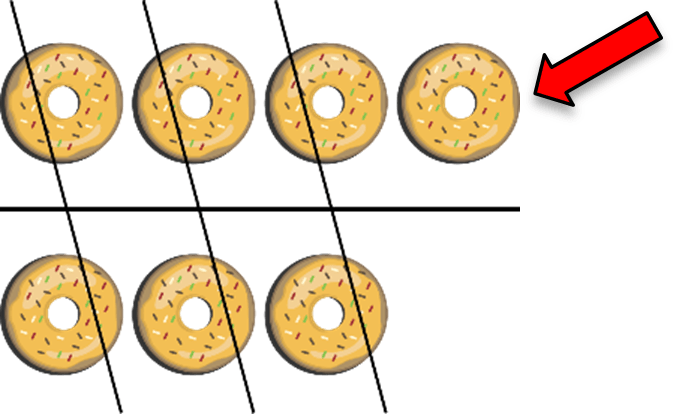• Let them replace the donuts with x:• Have the students talk with their partner about what happened with the exponents. The division canceled them out, or they may notice that now, the exponents are subtracted.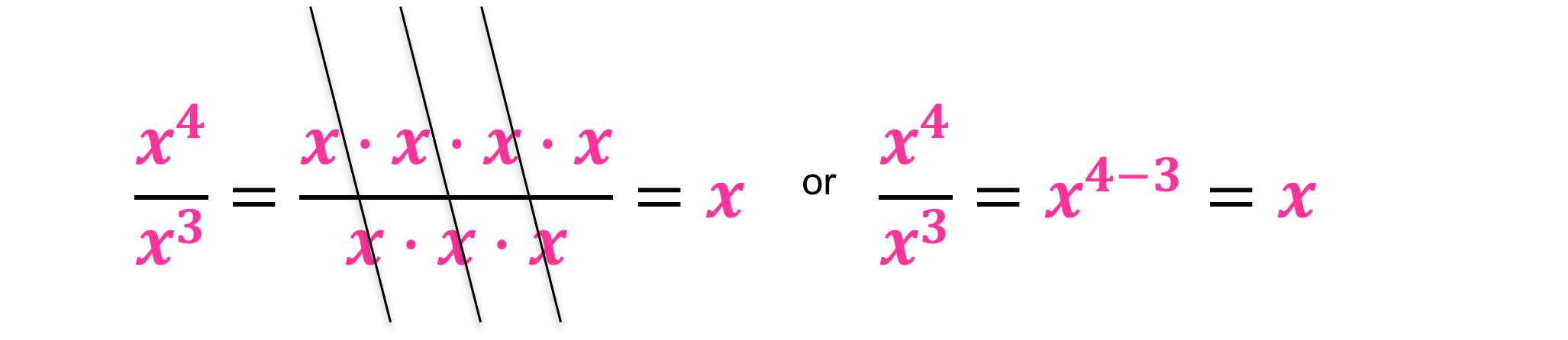Zero Exponent

• Emphasize that one donut stands for x
• Ask: “What will happen if the dividend and the divisor are the same? How would subtraction work?“
• Some students may be able to figure out how it is done. Their answer should look like the one below.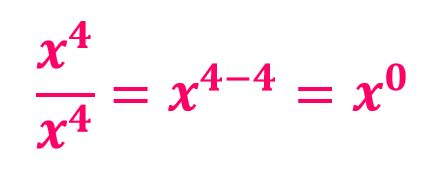• Ask: “So what does x⁰ mean?
• Well, if your students aren’t sure, have them model it. It would look like this:And this means 1 whole!

• If they still don’t quite understand, you can ask things like “What’s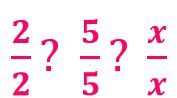These are all equal to 1.  Therefore, if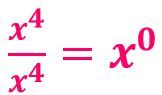and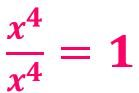, then we can say that x⁰ = 1.
• That helps prove anything that has a power of 0 is equal to 1, because looking at the donuts; they are all cancelled out! Remember, dividing anything by itself is ALWAYS equal to 1.

Negative Exponent

• Emphasize that one donut stands for x
• Ask: “What is the quotient of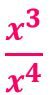”
• Students may come up with an answer using a model like the one below: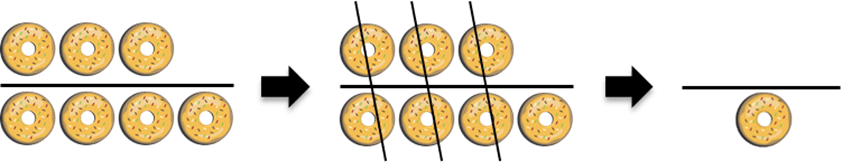• After learning the quotient rule, where exponents of the same base are subtracted, someone may show: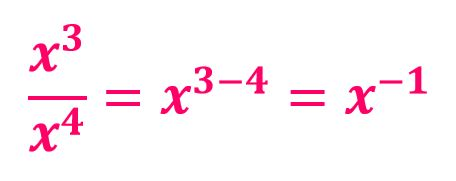• So what is a negative exponent?
•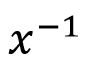is the same as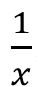.
• A negative exponent isn’t a negative number, it just flips the variable down to the denominator.1• Let the students try and figure out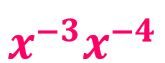which is the same as.
• Students may model it, or students may add the exponents, since the problem involves product of exponents.
• To model the expression, refer to the image below:• If other students used the product rule, since the given problem involves multiplication, they will most likely add the exponent and then later see that the answers match.
• Adding the exponents: – 3 + ( – 4) = – 7
• So,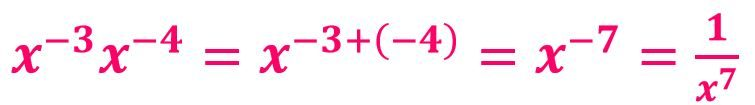• Another possible solution is: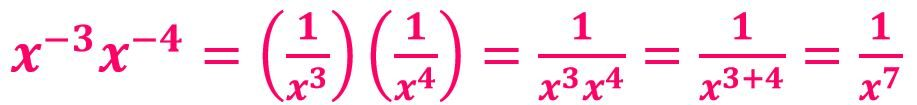After the discussion, it’s time to introduce the game.  It’s ok if the students don’t see all of the flips and patterns, because during the game, you can walk around and observe, help out and answer questions.

The games should reinforce the laws of exponents, and you can also let them continue to model it with tokens as they play, especially if they find it challenging to remember the rules for exponents.

Know and Apply the Properties of Integer Exponents Activity: Integer Exponent Card Game – General Instructions

• Tell the students that they will be playing a card game that is a little bit like War.
• There are 2 rounds:
• The first round involves the Product of Powers.
• The second round involves the Quotient of Powers.
• The goal of the game is to earn all cards. Whoever gets all the cards, or most of them, wins the game.
• To win the cards for each throw, they must show the expression with variables and exponents, and have the smallest product or quotient of powers (after either multiplying or dividing).
• They will use a normal deck of cards.
• The sign of the exponents depends on the color, number and face of the card.
• For this game:
• All RED cards have a negative value.
• All BLACK cards have a positive value.
• Number Cards
• All number cards are worth their spot or index value.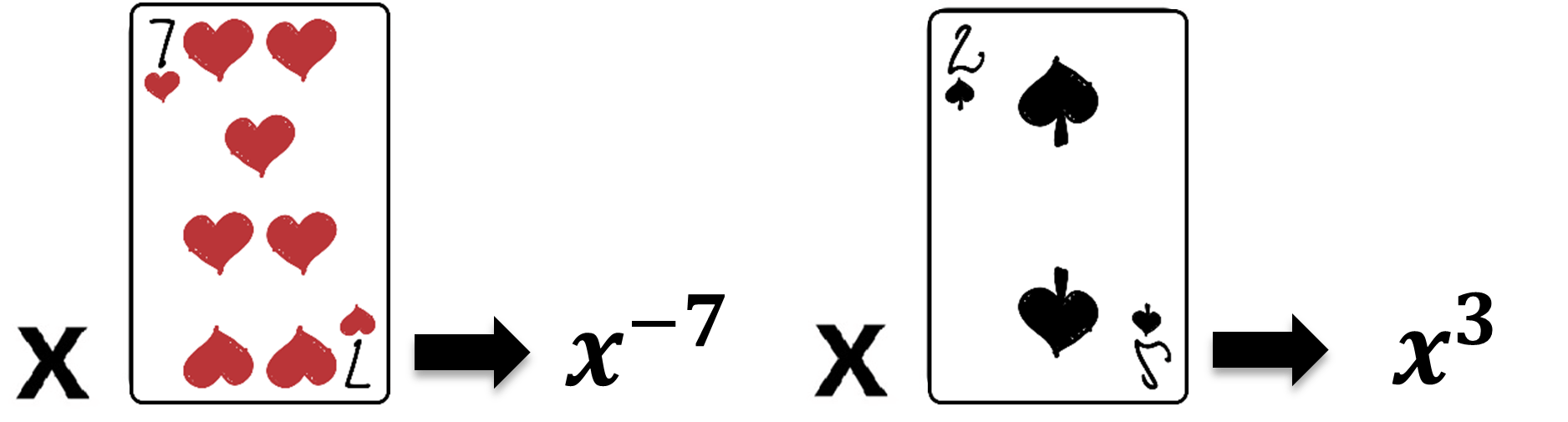• Face Cards
• Jack has a value of 11
• Queen has a value of 12
• King has a value of 13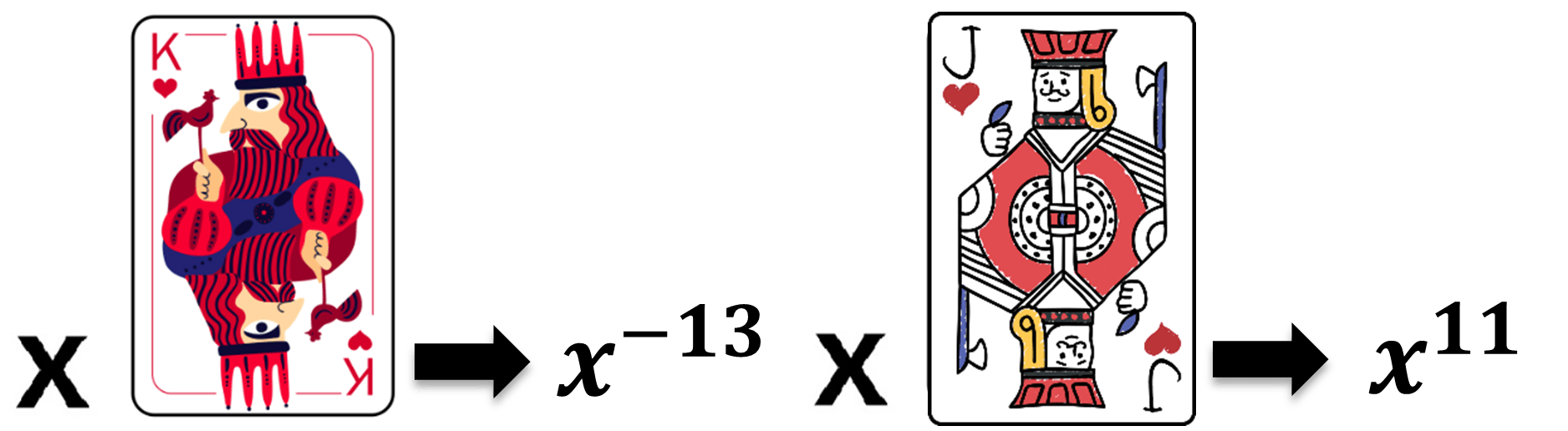• Divide the deck of cards evenly.
• Keep the pile face down.

Integer Exponent Card Game Round 1:  Product of Powers

1. Each partner has a product template.
2. Lay down your top 2 cards, each in the exponent spot.
4. In cases where the product of powers is negative, you may keep it as it is. There is no need to make it positive.
5. Whoever has the SMALLEST product of powers wins all those 4 cards.
6. Keep winning cards in a separate pile.
7. Play again.
8. The game continues until:
• There’s a tie- tie breaker, WAR, just play 2 cards face down and 2 cards face up (4 more cards each) and calculate the new product of powers battle.
• If someone gets a simplified answer equal to 1 – (this can be obtained if the resulting product of powers is zero), you take the winning pile of cards from your opponent.
• Someone who can get all the cards, or most of them, is the WINNER!
1. You can set a time limit of 10-15 minutes, so they have time to play the Quotient of Powers
1. See the images below for your reference: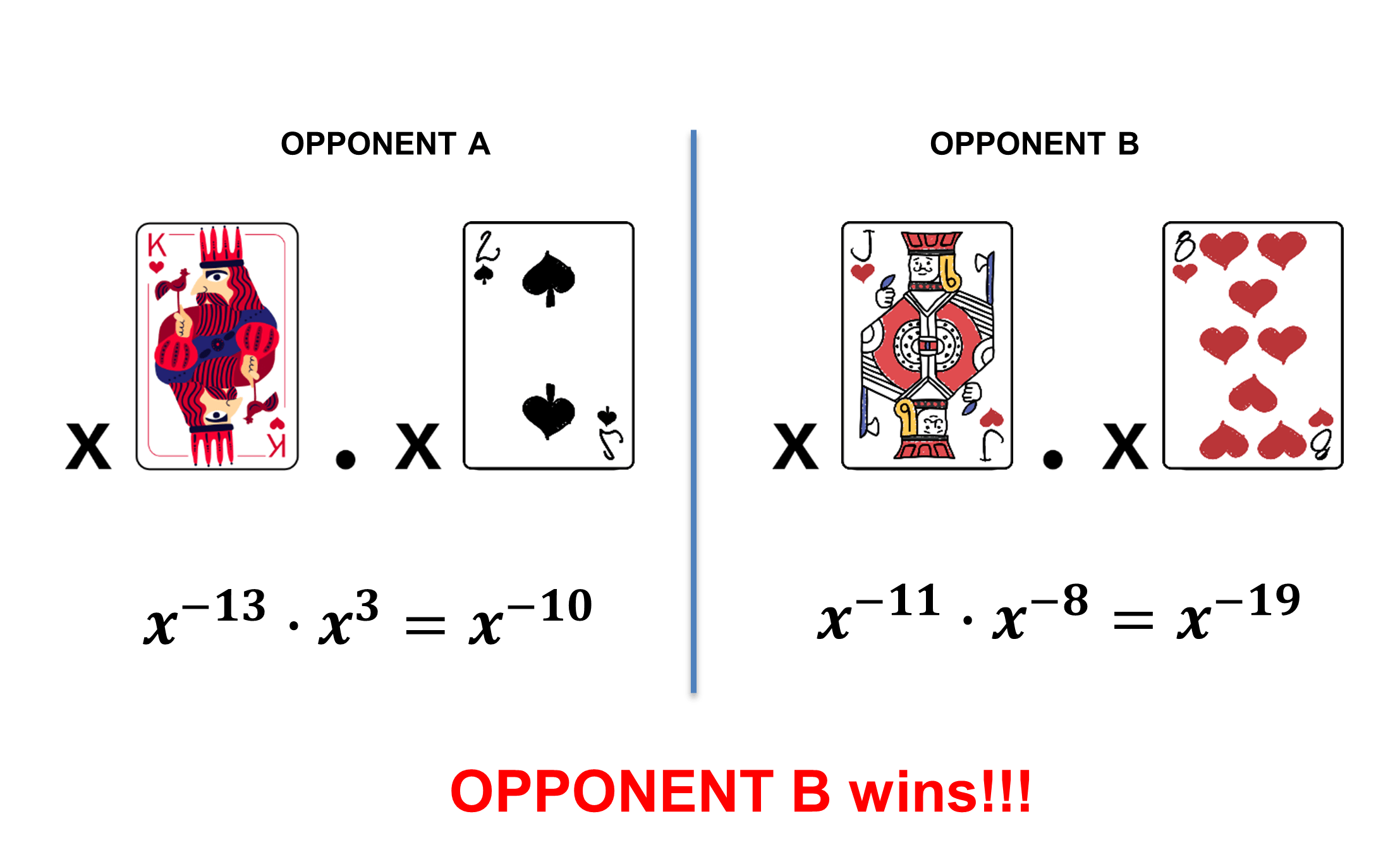Integer Exponent Card Game Round 2: Quotient of Powers

1. This has the same rules, but each partner has a quotient template in front of him/her.
2. Players lay down 2 cards, face up, write the expression, and solve the quotient of powers.
4. Whoever has the SMALLEST quotient of powers wins all those 4 cards.
5. In cases where the quotient of powers is negative, you may keep it as it is. There is no need to make it positive.
6. Keep winning cards in a separate pile.
7. Play again.
8. Play continues until:
• There’s a tie- tie breaker, WAR, just play 2 cards face down and 2 cards face up (4 more cards each) and calculate the new quotient of powers battle.
• If someone gets a simplified answer equal to 1 – (this can be obtained if the resulting quotient of powers is zero), you take the winning pile of cards from your opponent.
• Someone who can get all the cards, or most of them, is the WINNER!
1. See the images below for your reference: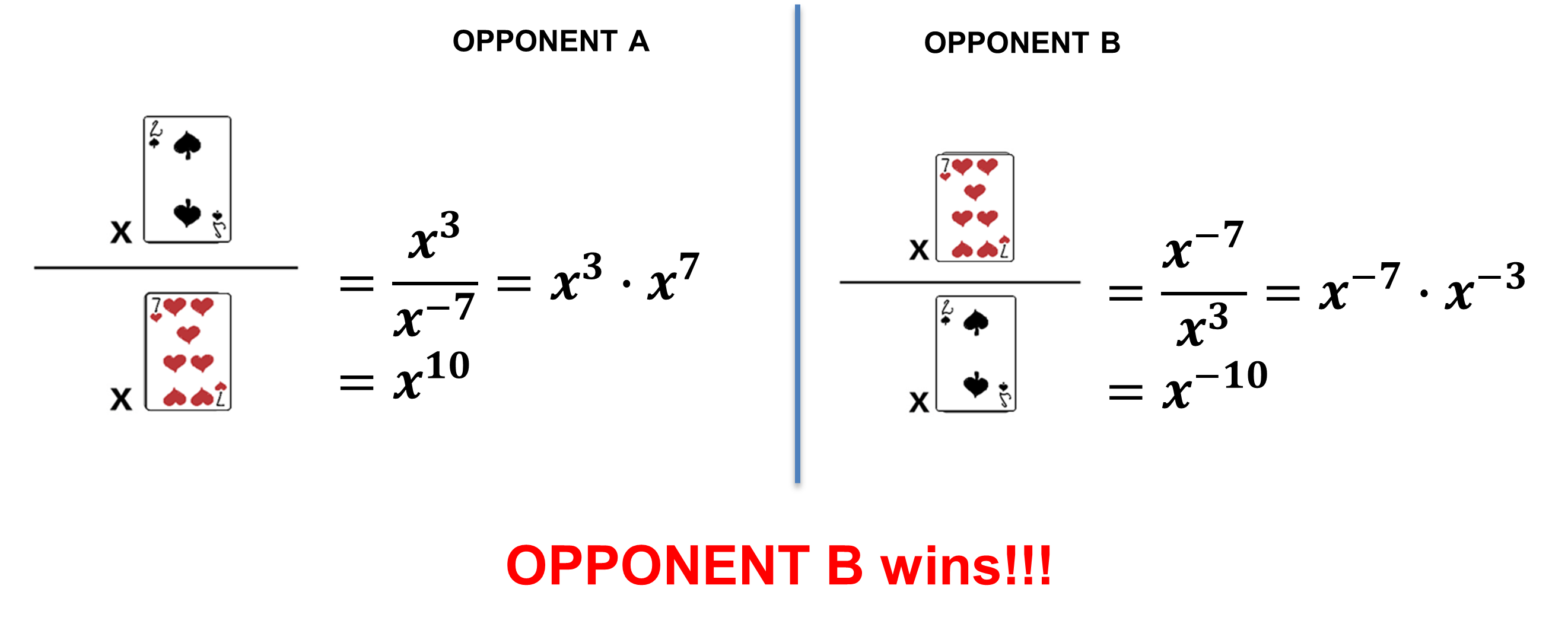Reflecting on the Game

Since the students are working in pairs, be sure to walk around, ask questions and listen to their ideas and conversations.

At the end of each round, have the students discuss what types of cards they were hoping to get when they laid them on the templates.

• “Did you want a red card or black card?”
• “When you were playing a card for the denominator of the quotient template, did you want black or red? Large or small?”

Students can discuss and answer these types of questions on the worksheet as well.

Use the expressions obtained by students in the game and let them explain how they won a round. You may also ask them what questions they came across with while playing the game.

Ask how the game helped them understand negative exponents.

Extensions

Make a foldable showing each of the exponent rules. Cut a square of paper, fold each corner into the middle to make a smaller square.  On the outside flaps, write an expression involving product or quotient of powers, then lift that flap and show how to solve it.

Desmos Activity Builder

Make a chart showing patterns from exponents when multiplied and/or divided.

Note to designer:  Place quotients as fractions with a top and a bottom, like this(not a backslash)